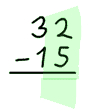# Subtraction by "Regrouping"

To subtract numbers with more than one digit:

• write down the larger number first and the smaller number directly below it
making sure to line up the columns!
• then do subtractions one column at a time like this (press play button):

## Smaller Number - Bigger NumberBut what if a column has a smaller number on the top?

How do we do 2 − 5 ?

The Solution: Make the "2" larger by "regrouping" (press arrow button):

images/moving-tens.js

In 32:

• the "3" means 3 tens,
• the "2" means 2 ones,

So we:

• reduce the 3 by 1
• increase the 2 by 10

And we get:

• 2 tens,
• 12 ones

And here is how to do it with a pencil and paper:

We use this method whenever a column has a smaller number on top, as in this example:

## Zero

But we can't regroup from zero ...

But we can make a "0" into a "10" by regrouping it first, them carry on normally, like this:

Now, you can practice with these Subtraction Worksheets

1600, 1601, 3412, 3413, 1602, 1603, 3414, 3415, 2948